## Python: Introduction IVIt has been a while back I wrote about Python Introduction I, II and III. Today, I am going to complete the last part of the introduction, the NumPy. Months ago during my Python’s self-learning time, I wrote about NumPy, here is the link.

### NumPy

It is an alternative to Python List, the NumPy array helps us to solve problems dealing with Python List’s operations. Calculations on Python Lists cannot be done in the same way we do for two integers or strings. This package needs to be installed before we can import and use it.

In my blog above, I wrote about the behaviour of the NumPy Array. It does not allow different types of elements in the array. When a NumPy Array is built, element’s data type changed to end up with a homogeneous list. Supposed, the list contains a string, number and Boolean, now it changes to all string format, for an example.

Also, the operator “+”, “-” and etc which we used along with Python List, are different in NumPy Array. Refer below for an example:

```py_list = [1,2,3]
numpy_array = np.array([1,2,3])
py_list + py_list
numpy_array + numpy_array
```

First output shows the two lists are merged or combined together into a single list. Second output shows an array returns an output of addition of those numbers. The screenshot below shows the result which I used Jupyter Notebook to execute.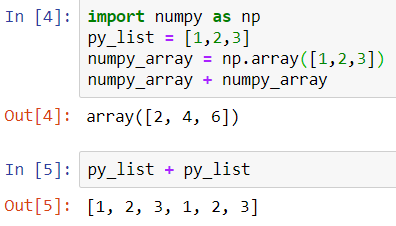Whatever that it has covered in the link above is good enough to give us a basic understanding of Numpy. If you wish to learn more, there is another link I found from the Medium which we can refer to.

### NumPy Subsetting

Specifically for NumPy, there is a way of doing list subsetting, using an array of Boolean. Example below shows how we can get all the BMI values above 23. Refer to the example from DataCamp,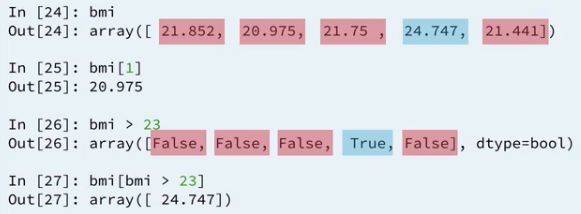First result returns as Boolean, True if BMI value is above 23. Then, you can use this Boolean array inside a square bracket to do the subsetting. When the Boolean’s value is True, it selects its value.

In short, it is using the result of the comparison to make a selection of data.

### 2D NumPy Array

I covered the 2D NumPy Array in this link, where it shows how to declare a 2D NumPy Array and how does it work in subsetting, indexing, slicing and perform math operations.

### NumPy: Basic Statistics

You can generate summary statistics of the data using NumPy. Python NumPy has few useful statistical functions which can be used for analytics. It includes finding min, max, average, standard deviation, variance and etc. from a given elements in the array. Refer to my write up on this basic statistics in this link.

## Data Types : StatisticsTwo main data types in the Satistics, the qualitative data and the quantitative data. In the previous topic, we discussed about the “Level of Data Measurement” in which we talked about nominal, ordinal, interval and ratio. How does these measurements can be related to qualitative and quantitative data.

The qualitative data is the nominal and ordinal measurements which describe a “feature” of a data object. Meanwhile, the quantitative data refers to data can be counted or measured by numbers. The quantitative data is the interval and ratio measurements.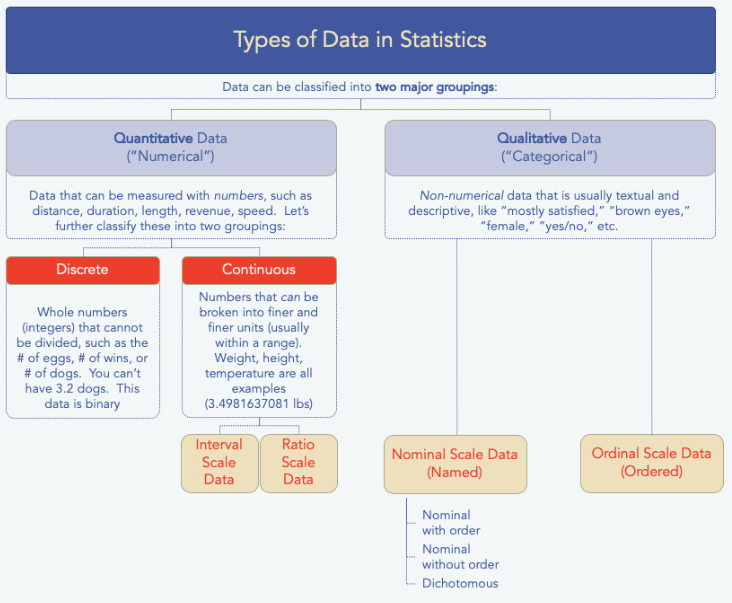More examples to distinguish the qualitative data and quantitative data as below: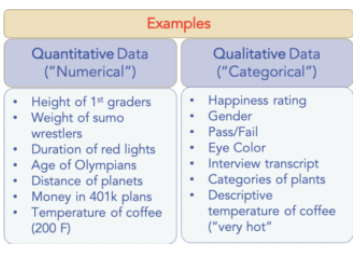And, further discussion on the qualitative data where it has a sub level of data types called discrete and continuous. Both have differences in few areas, see the table below: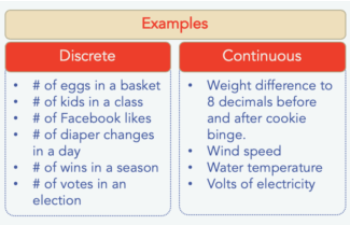Discrete data is a whole number (integer) and it cannot be subdivided into smaller and smaller parts.

Continuous data continues on and on and on.

## Levels of Data Measurement : StatisticsLast week, during my 2nd class in Business Intelligence, a statistics topic on levels of measurement was being discussed. The lecturer tried her very best to explain to us the differences between each of the levels.

## Nominal, Ordinal, Interval or Ratio.

In statistics, there are four levels of data measurement, nominal, ordinal, interval and ratio (and sometimes, the interval and ratio are called in other terms such as continuous and scale).

I think this is important for researchers to understand this theory part of statistics to determine which statistic analysis suitable for their problem statements. And, for students like me, I think it is good enough if I can differentiate them as I was told, the exam paper would not ask us to differentiate but theoretically, we have to understand what each of them is.

There are a number of statistics’ articles online which explained it and I found the website called, http://www.mymarketresearchmethods.com gave me a better understanding. You can refer to the link below for the write-up and I will explain a bit here too.

It is quite easily to distinguish the nominal and ordinal measurements.

## Nominal & Ordinal

First level of measurement is nominal. The numbers in the variable are used only to classify the data. The words, letters, and alpha-numeric symbols can be used as the values or numbers in the variable (without quantitative value). Best example is gender, male or female.

Nonimal data can be in ordered or no ordered such as gender. Ordered nominal data can be something like, cold, warm, hot and very hot.

Second level of measurement is ordinal. With ordinal scales, the order of the values is what is important and significant, but the differences between each one is not really known. Examples is the ranking No.1, No.2 and No.3 for students’ score, with highest score is No.1 follows by second highest score gets No.2.

However, I get a bit confused because the above mentioned, “the differences between each one is not really known”. But scores and ranks did tell the differences, unless we use exam grade such as grade A+, A and A-. Do you agree with this example?

Maybe, I shall follow what the website says, the satisfaction level, it cannot quantify–how much better it is.

## Interval & Ratio

The third level of measurement is interval. Interval scales are numeric scales in which we know both the classification, order and the exact differences between the values. I picked up the explanation from the same website.

Like the others, you can remember the key points of an “interval scale” pretty easily. “Interval” itself means “space in between,” which is the important thing to remember–interval scales not only tell us about order, but also about the value between each item.

For example, the difference between 60 and 50 degrees is a measurable 10 degrees, as is the difference between 80 and 70 degrees.

Here’s the problem with interval scales: they don’t have a “true zero.” For example, there is no such thing as “no temperature,” at least not with celsius. In the case of interval scales, zero doesn’t mean the absence of value, but is actually another number used on the scale, like 0 degrees celsius. Negative numbers also have meaning. Without a true zero, it is impossible to compute ratios. With interval data, we can add and subtract, but cannot multiply or divide.

Consider this: 10 degrees C + 10 degrees C = 20 degrees C. No problem there. 20 degrees C is not twice as hot as 10 degrees C, however, because there is no such thing as “no temperature” when it comes to the Celsius scale. When converted to Fahrenheit, it’s clear: 10C=50F and 20C=68F, which is clearly not twice as hot.

The fourth level of measurement is the ratio. Ratio tells us about the order, they tell us the exact value between units, AND they also have an absolute zero–which allows for a wide range of both descriptive and inferential statistics to be applied. Good examples of ratio variables include height, weight, and duration. These variables can be meaningfully added, subtracted, multiplied, divided (ratios).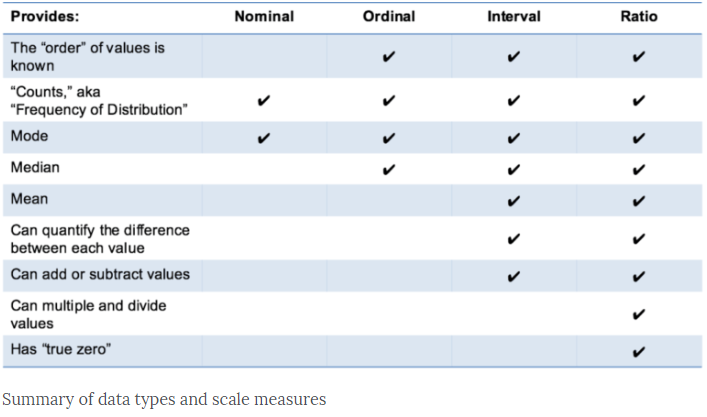## Day 23: Python Basic Statistics

One of the interesting exercises for Python so far, I want to share my source code for the question I extracted from the online learning website. The datasets for heights and positions are pre-randomized from the website.

• Convert `heights` and `positions`, which are regular lists, to numpy arrays. Call them `np_heights` and `np_positions`.
• Extract all the heights of the goalkeepers. You can use a little trick here: use `np_positions == 'GK'` as an index for `np_heights`. Assign the result to `gk_heights`.
• Extract all the heights of all the other players. This time use `np_positions != 'GK'` as an index for `np_heights`. Assign the result to `other_heights`.
• Print out the median height of the goalkeepers using `np.median()`. Replace `None` with the correct code.
• Do the same for the other players. Print out their median height. Replace `None` with the correct code.
```# Import numpy
import numpy as np

# Convert positions and heights to numpy arrays: np_positions, np_heights
np_heights = np.array(heights)
np_positions = np.array(positions)

# Heights of the goalkeepers: gk_heights
gk_heights = np_heights[np_positions == 'GK']

# Heights of the other players: other_heights
other_heights = np_heights[np_positions != 'GK']

# Print out the median height of goalkeepers. Replace 'None'
print("Median height of goalkeepers: " + str(np.median(gk_heights)))

# Print out the median height of other players. Replace 'None'
print("Median height of other players: " + str(np.median(other_heights)))
```

The output of the above source code execution is,

Median height of goalkeepers: 188.0
Median height of other players: 181.0

## Day 23: Python NumPy – Basic Statistics

Python NumPy has few useful statistical functions which can be used for analytics. It includes finding min, max, average, standard deviation, variance and etc. from a given elements in the array. Some basic examples I captured from the online learning website as below: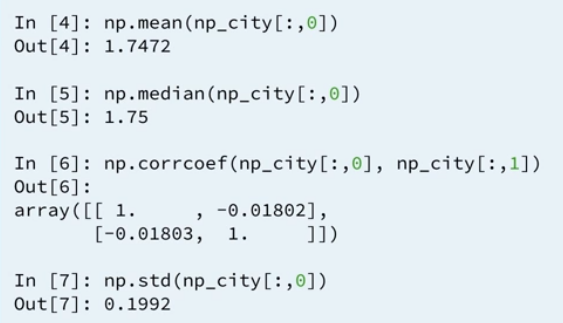I searched further from the other tutorial website, it gives a basic understanding on what these terms are and a sample codes and its output.

Mean numpy.mean()
– Sum of elements along an axis divided by the number of elements.

```import numpy as np
a = np.array([[1,2,3],[3,4,5],[4,5,6]])

print 'Our array is:'
print a
print '\n'

print 'Applying mean() function:'
print np.mean(a)
print '\n'

print 'Applying mean() function along axis 0:'
print np.mean(a, axis = 0)
print '\n'

print 'Applying mean() function along axis 1:'
print np.mean(a, axis = 1)
```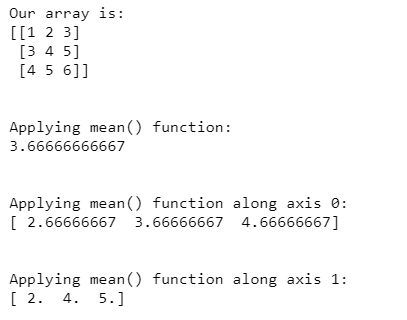Axis 0 means adding the values vertically.
Axis 1 means adding the values vertically.

Median numpy.median()
– Defined as the value separating the higher half of a data sample from the lower half. (Combine the element before defining).

```import numpy as np
a = np.array([[30,65,70],[80,95,10],[50,90,60]])

print 'Our array is:'
print a
print '\n'

print 'Applying median() function:'
print np.median(a)
print '\n'

print 'Applying median() function along axis 0:'
print np.median(a, axis = 0)
print '\n'

print 'Applying median() function along axis 1:'
print np.median(a, axis = 1)
```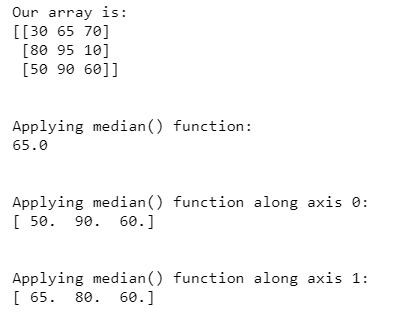Standard Deviation numpy.std()
– The square root of the average of squared deviations from means.
– Formula:std = sqrt(mean(abs(x – x.mean())**2))

Example of numpy.std():

```import numpy as np
print np.std([1,2,3,4])

# Output:
1.1180339887498949
```

Variance numpy.var()
– The average of squared deviations.
– The standard deviation is the square root of variance.
– Formula: mean(abs(x – x.mean())**2)

Example of numpy.var():

```import numpy as np
print np.var([1,2,3,4])

# Output:
1.25
```

We can use this information to generate randomized sample data. Based on an example from DataCamp, the screenshot below shows how it uses the distribution mean’s value, standard deviation’s value and sample size to generate data.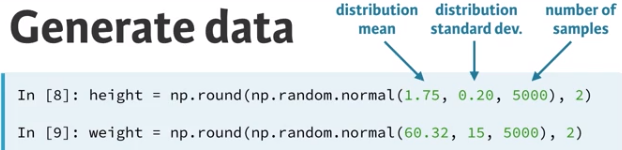Other statistical functions such as percentile, correlations and etc. will be covered when I have some examples on this functions.

Summary of the day:

• Numpy statistical functions
• numpy.mean()
• numpy.median()
• numpy.std()
• numpy.var()